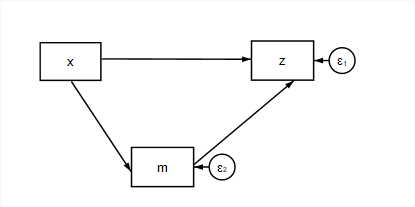# MPlus Mediation

## Intro

The idea of mediation is that one variable (an independent variable) may influence another variable (a response) through it’s influence on a third variable (the mediator). In the following diagram, $$x$$ is the independent variable, $$z$$ is the response variable, and $$m$$ is a mediating variable.Simple mediation

When all of the relations (direct effects) in our model are linear regressions, in addition to the coefficients that characterize

$z = \beta_0 + \beta_1 x + \beta_2 m + \epsilon_z$ $m = \beta_4 + \beta_5 x + \epsilon_m$

we are often interested in estimating the indirect effect of x on z, $$\beta_2 \beta_5$$, the total effect of x on z, $$(\beta_2 \beta_5) + \beta_1$$, and the proportion of the total effect that is mediated by m, $$\frac{\beta_2 \beta_5}{(\beta_2 \beta_5) + \beta_1}$$.

## SEM Estimation

The underlying model can be conveniently estimated through structural equations modeling. Using MPlus, not only can the software estimate the underlying model but it can also be used to give us estimates of the indirect effects, total effects, and the proportion mediated.

### Model Indirect

The underlying model is composed of two regressions. If we add a MODEL INDIRECT command, and specify the relation between x and z as ind, this will give us the underlying coefficients, direct, indirect, and total effects.

MODEL:
z on x m;
m on x;

MODEL INDIRECT:
z ind x;

yields the usual model results like

MODEL RESULTS

Two-Tailed
Estimate       S.E.  Est./S.E.    P-Value

Z        ON
X                  1.922      0.286      6.731      0.000
M                  0.013      0.071      0.176      0.860

M        ON
X                  3.977      0.037    107.459      0.000

Means
X                  0.075      0.070      1.083      0.279

Intercepts
M                  0.047      0.037      1.300      0.193
Z                 -0.003      0.037     -0.083      0.934

Variances
X                  0.968      0.097     10.000      0.000

Residual Variances
M                  0.265      0.027     10.000      0.000
Z                  0.269      0.027     10.000      0.000

as well as the additional effects

TOTAL, TOTAL INDIRECT, SPECIFIC INDIRECT, AND DIRECT EFFECTS

Two-Tailed
Estimate       S.E.  Est./S.E.    P-Value

Effects from X to Z

Total                1.972      0.037     52.919      0.000
Total indirect       0.050      0.283      0.176      0.860

Specific indirect 1
Z
M
X                  0.050      0.283      0.176      0.860

Direct
Z
X                  1.922      0.286      6.731      0.000

In this particular example, there are no measurable indirect effects, because there is no direct effect of m on z!

### Model Constraint

It is also possible to estimate indirect and total effects by writing our own “model constraints” (a misnomer in this case, because nothing is actually constrained). The advantage in writing your own constraints is that there is usually less output to wade through, and you can also estimate the proportion of mediation.

To achieve this, we need to label our regression coefficients in the original model command.

MODEL:
z on (zx);
z on m (zm);
m on x (mx);

MODEL CONSTRAINT:
NEW(zmx TOTAL prop); ! declare additional variables;
zmx = zm*mx;         ! Indirect effect of x on z via M;
TOTAL = zm*mx + zx;  ! Total effect of X on Y;
prop = zmx/(TOTAL);

In addition to the model results like before, at the end of the MODEL RESULTS section we also get

New/Additional Parameters
ZMX                0.050      0.283      0.176      0.860
TOTAL              1.972      0.037     52.919      0.000
PROP               0.025      0.144      0.176      0.860